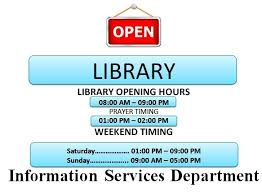It looks like you're using Internet Explorer 11 or older. This website works best with modern browsers such as the latest versions of Chrome, Firefox, Safari, and Edge. If you continue with this browser, you may see unexpected results.

# Network Analysis: Home

Network Analysis methods is a group of special analytical methods that are used in case where it is necessary to analyze and optimize a network of inteconnected and related elements that have some connection between one another.

## Level of Study

Undergraduate## Library Timing## Course Description & Objectives

Course description: This course introduce Inductors and capacitors as energy storage elements, natural response, transient response and steady state response of first order circuits. Current and voltage transients. RLC circuits with DC and AC excitation. Resonant circuits: series and parallel resonance in AC circuit, Q-Factor. Introduction to phasor representation of alternating voltage and current. Methods of AC Analysis. AC Network Theorems. Single-phase circuit analysis, power in single phase circuits and different methods of its measurements. Star-delta transformation for DC and AC circuits. Two-port networks and their interconnections. Filters: the Low-Pass, High-Pass, Band-Pass and Band-Reject Filters. Introduction to BODE plots. Introduction to Laplace transform and application of Laplace transform in circuit analysis.

Course objectives: This is a second course in Electrical Circuit Theory. This course covers various aspects of electrical networks. In this course, Electrical Engineering students will be taught to analyze RLC circuit in time domain, in phasor form and in frequency domain. Student will learn power transmission in single phase system, their power measurement methods and power factor correction for efficient transmission of power. Students will analyze circuits using network theorems in frequency domain. Student will learn about different filters. Students will be given the concept to formulate equations and choose among the different methods to analyze an electrical network.
The main Course Learning Outcomes (CLOs) of the subject are given below:

• CLO1: Interpret the behavior of energy storing elements and their transient response analysis. Explain Time domain, Phasor and Frequency domain response of RLC circuits. (C2)
• CLO2: Manipulate the effect of power transfer, power dissipation and power factor on power transmission. (C3)
• CLO3: Compare and understand the AC circuits using the network laws and theorems. (C4)
• CLO4: Differentiate between different filters. (P3)